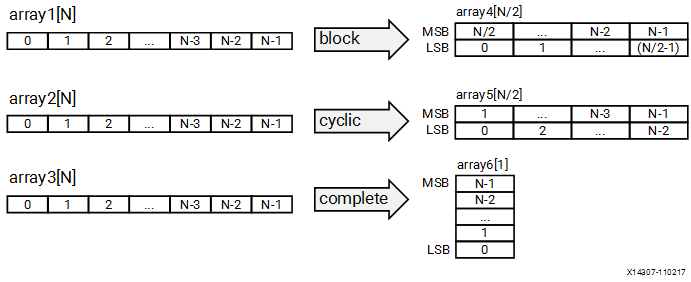xcl_array_reshape - 2021.2 English

Vitis Unified Software Platform Documentation: Application Acceleration Development (UG1393)

Document ID
UG1393
ft:locale
English (United States)
Release Date
2021-12-15
Version
2021.2 English

Description

Important: Array variables only accept one attribute. While the XCL_ARRAY_RESHAPE attribute does support multi-dimensional arrays, you can only reshape one dimension of the array with a single attribute.

This attribute combines array partitioning with vertical array mapping.

The XCL_ARRAY_RESHAPE attribute combines the effect of XCL_ARRAY_PARTITION, breaking an array into smaller arrays, and concatenating elements of arrays by increasing bit-widths. This reduces the number of block RAM consumed while providing parallel access to the data. This attribute creates a new array with fewer elements but with greater bit-width, allowing more data to be accessed in a single clock cycle.

Given the following code:

void foo (...) {
int array1[N] __attribute__((xcl_array_reshape(block, 2, 1)));
int array2[N] __attribute__((xcl_array_reshape(cycle, 2, 1)));
int array3[N] __attribute__((xcl_array_reshape(complete, 1)));
...
}

The ARRAY_RESHAPE attribute transforms the arrays into the form shown in the following figure.

Figure 1. ARRAY_RESHAPESyntax

Place the attribute with the definition of the array variable.

__attribute__((xcl_array_reshape(<type>,<factor>,
<dimension>)))

Where:

• <type>: Specifies one of the following partition types:
• cyclic: Cyclic partitioning is the implementation of an array as a set of smaller physical memories that can be accessed simultaneously by the logic in the compute unit. The array is partitioned cyclically by putting one element into each memory before coming back to the first memory to repeat the cycle until the array is fully partitioned.
• block: Block partitioning is the physical implementation of an array as a set of smaller memories that can be accessed simultaneously by the logic inside the compute unit. In this case, each memory block is filled with elements from the array before moving on to the next memory.
• complete: Complete partitioning decomposes the array into individual elements. For a one-dimensional array, this corresponds to resolving a memory into individual registers. The default <type> is complete.
• <factor>: For cyclic type partitioning, the <factor> specifies how many physical memories to partition the original array into in the kernel code. For Block type partitioning, the <factor> specifies the number of elements from the original array to store in each physical memory.
Important: For complete type partitioning, the <factor> should not be specified.
• <dimension>: Specifies which array dimension to partition. Specified as an integer from 1 to <N>. The Vitis core development kit supports arrays of <N> dimensions and can partition the array on any single dimension.

Example 1

Reshapes (partition and maps) an 8-bit array with 17 elements, AB, into a new 32-bit array with five elements using block mapping.

int AB __attribute__((xcl_array_reshape(block,4,1)));
Tip: A <factor> of 4 indicates that the array should be divided into four. As a result, the 17 elements are reshaped into an array of five elements, with four times the bit-width. In this case, the last element, AB, is mapped to the lower eight bits of the fifth element, and the rest of the fifth element is empty.

Example 2

Reshapes the two-dimensional array AB into a new array of dimension , in which dimension 2 has twice the bit-width:

int AB __attribute__((xcl_array_reshape(block,2,2)));

Example 3

Reshapes the three-dimensional 8-bit array, AB in function foo, into a new single element array (a register), 128-bits wide (4×2×2×8):

int AB __attribute__((xcl_array_reshape(complete,0)));
Tip: A <dimension> of 0 means to reshape all dimensions of the array.